Download Presentation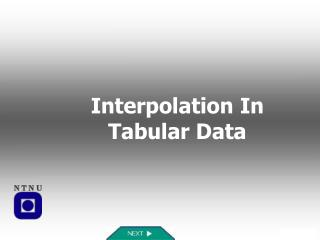Interpolation In Tabular Data

# Interpolation In Tabular Data - PowerPoint PPT Presentation

Interpolation In Tabular Data. Home. HOME. Introduction. Subroutine Subprograms. Lagrange’s Interpolation Formula. Programming Exercise. Resources. Useful Info. Quiz. Learning Objectives. Learning Objectives in this module : Review of methods for computer-aided interpolationI am the owner, or an agent authorized to act on behalf of the owner, of the copyrighted work described.
Download Presentation## Interpolation In Tabular Data

An Image/Link below is provided (as is) to download presentation

Download Policy: Content on the Website is provided to you AS IS for your information and personal use and may not be sold / licensed / shared on other websites without getting consent from its author.While downloading, if for some reason you are not able to download a presentation, the publisher may have deleted the file from their server.

- - - - - - - - - - - - - - - - - - - - - - - - - - E N D - - - - - - - - - - - - - - - - - - - - - - - - - -
Presentation Transcript### Interpolation In Tabular Data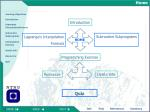Home

HOME

Introduction

Subroutine Subprograms

Lagrange’s Interpolation

Formula

Programming Exercise

Resources

Useful Info

QuizLearningObjectives

Learning Objectives in this module:

• Review of methods for computer-aided interpolation
• Develop problem solution skills using computers and numerical methods
• Develop programming skills using FORTRAN
• New FORTRAN elements in this module:
• use of subroutines
• working with arrays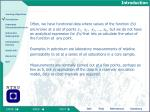Introduction

Often, we have functional data where values of the function f(x)

are known at a set of points x1 , x2 , x3 , ..., xN , but we do not have an analytical expression for f(x) that lets us calculate the value of the function at any point.

Examples in petroleum are laboratory measurements of relative

permeability to oil at a series of oil saturations in a core sample.

Measurements are normally carried out at a few points, perhaps as few as 5, but in application of the data in a reservoir simulation model, data at close intervals are required.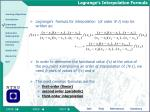Lagrange’s Interpolation Formula
• Lagrange’s formula for interpolation (of order N-1) may be written as:
• In order to determine the functional value f(x) at the value of the argument x employing an order of interpolation of (N-1), we need N pairs of values of f(x) and x.
• The most common formulas are the
• first-order (linear)
• second order (parabolic)
• third-order interpolation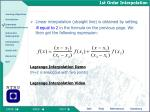1st Order Interpolation
• Linear interpolation (straight line) is obtained by setting

N equal to 2 in the formula on the previous page. We then get the following expression:

Lagrange Interpolation Demo

(N=2 is analogous with two points)

Lagrange Interpolation Video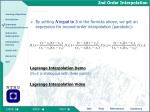2nd Order Interpolation
• By setting N equal to 3 in the formula above, we get an expression for second-order interpolation (parabolic):

Lagrange Interpolation Demo

(N=3 is analogous with three points)

Lagrange Interpolation Video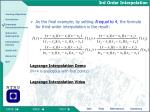3rd Order Interpolation
• As the final example; by setting N equal to 4, the formula for third-order interpolation is the result:

Lagrange Interpolation Demo

(N=4 is analogous with four points)

Lagrange Interpolation Video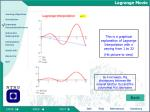Lagrange Movie

This is a graphical explanation of Lagrange Interpolation with n varying from 1 to 32

(Hit picture to view)

As n increases, the discrepancy between the original function f(x) and the polynomial P(x) decreases

BackSubroutine Subprograms

In this module you will use Subroutine Subprograms

A subroutine subprogram has the same overall structure as a function subprogram except that the first statement is a subroutine statement rather than a function statement

A subroutine is accessed by means of a call statement, which gives the name of the subroutine and a list of arguments which are used to transfer information between the main program and the subroutine:

call “name” (arg1,arg2,..............)

Again we’ll proceed with an easy example

More..

ExampleProgram

A program that calculates the mean of five numbers:

PROGRAM MEAN

REAL A1, A2, A3, A4, A5, AV

WRITE(*,*) ’Input five real numbers:’

READ(*,*)A1, A2, A3, A4, A5

CALL AVRAGE(A1, A2, A3, A4, A5, AV)

WRITE(*,100) AV

100 FORMAT(’Average of the five numbers is:’,1X,F10.4)

STOP

END

The AVRAGE subroutine Here

Full ProgramProgram

SUBROUTINE AVRAGE(X1, X2, X3, X4, X5, XBAR)

REAL X1, X2, X3, X4, X5, SUM, XBAR

SUM = X1 + X2 + X3 + X4 + X5

XBAR=SUM/5.0

RETURN

END

• This is the AVRAGE subroutine subprogram. It is located after the main program, much the same as the function subprogram you have learnt in an earlier module
• The full mean.f Fortran Program as it would look in emacshere

NotesNotes
• The actual arguments, A1, A2, A3, A4, A5, AV replace the dummy arguments X1, X2, X3, X4, X5, XBAR which appear in the subroutine definition. They must match in number, type and order
• The variables X1, X2, X3, X4, X5, XBAR are LOCAL to the subprogram. Thus, when writing a subprogram or a main program we need not be concerned that names used in one program unit might clash with those in another program unit.
• This is one reason why it then becomes possible to write libraries of useful subprograms, functions and subroutines, which can subsequently be used in other programs of yours or other people's
• As opposed to function subprograms, subroutine subprograms can return several valuesInterpolation Exercise
• Make a FORTRAN program that uses Lagrange’s formula for interpolation in order to find a value of the function f(x) corresponding to a value xin a table .
• The program should be made so that the order of interpolation, M, is an input parameter. The program shall consist of a main program that first reads the table values. These include the number of table entries, N, and values FXT(I) and XT(I), I=1,...,N. (table should be in ascending order). Then, it should read single values of X and M, and carry out interpolation of order M in order to determine the FX-value.
• Then, the subroutineLAGRANGE(X,FX,M,N,XT,FXT) is called for interpolation. In order to apply the Lagrange formula, it first needs to locate the X-value in the input table.
• It may be convenient to make a separate subroutine for this, LOCATE(X,I1,N,XT), that returns position I1 (ie. X is larger than XT(I1) and less than XT(I1+1)). The LAGRANGE routine then carries out the interpolation and returns the result to the main program.

More...

NextInterpolation Exercise
• In this exercise, we use first-, second- and third-order interpolation. After carrying out the interpolation, the main program will write the interpolated values as well as the input values in a table. The main program should check that M<N. If not, a message should be written and the runs should be stopped.
• The program should be run for two different data sets:

Data Set 1

Data Set 2Data Set 1

Here, the function f(x)=x3

has been used to create the

following table:

Read the input table and use the FORTRAN program to find values for f(x) at x=(0.1; 0.3; 0.5; 0.7; 0.9) using 1.-, 2.- and 3.-order Lagrange interpolation. The program should print the following table with interpolated values:

More..

Resources

Useful InfoData Set 2
• The following values for relative permeability (kro) vs. oil saturation (So) for oil has been measured on a core sample in the laboratory:
• Read the table and use the FORTRAN program to generate a new table with 5% intervals in oil saturation (So=0.2; 0.25; 0.3...).

Make these new tables using 1.-, 2.- and 3.-order Lagrange interpolation. Plot the new tables using Excel.

More

Resources

Useful InfoResources

Introduction to Fortran

Fortran Template here

The whole exercise in a printable format here

Web sites

• Numerical Recipes In Fortran
• Fortran Tutorial
• Professional Programmer's Guide to Fortran77
• Programming in Fortran77

Useful InfoUseful Info

For the Interpolation program:

• To get the input table in ascending order, use the sorting subroutine you made in the previous module
• For the LOCATE subroutine, take a look in the textbook, page 110 or visit:Ch. 3.4: How to search an ordered table
• This program is overall rather big, though it should consist of several smaller program parts, so keep track of your DO loops, labels and variables

ResourcesQuiz
• This section includes a quiz on the topics covered by this module.
• The quiz is meant as a control to see if you have learned some of the most important features
• Hit object to start quiz
• (Depending on your connection, this may take a few seconds...)General informationFAQ
• No questions have been posted yet. However, when questions are asked they will be posted here.
• Remember, if something is unclear to you, it is a good chance that there are more people that have the same question

For more general questions and definitions try these

Dataleksikon

Webopedia

Schlumberger Oilfield GlossaryReferences
• W. H. Preuss, et al., “Numerical Recipes in Fortran”, 2nd edition

Cambridge University Press, 1992

• References to the textbook :

Lagrange interpolation: page 99

Finding table entries: page 110Summary

Subsequent to this module you should...

• be familiar with the Lagranges Interpolation Formula
• know how to efficiently use subroutines
• have obtained a better understanding on solving problems in FortranProgram mean.f

The subroutine AVRAGE is called here

Commenarty lines; will not be treated as a part of the program when run

Main program

The order of the variables called must be equal to the order in the subroutine, though they need not have the same name

Subroutine

Click exclamation marks for notes

Back

See the Program RunRun Program

Compiling mean.f

Running proram

Inputting five real numbers

Getting the result of the calculation

Back